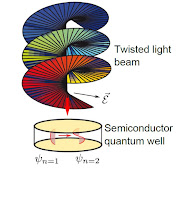### Twisted-light-induced transitions in quantum wells

One of the most basic semiconductor heterostructures clean rooms all over the world routinely build are quantum wells (QW). They comprise a thin layer of a small bandgap semiconductor sandwiched between larger bandgap materials and are thus able to confine electrons to two dimensions. Besides their technological importance they have featured prominently over and over again in some of the most influential experiments in condensed matter physics, from quantum Hall effects to recently discovered topological insulators.

Post contributed by:
Björn Sbierski,
Freie Universität Berlin,
Germany

Blog post based on the journal article "Twisted-light-induced inter-subband transitions in quantum wells at normal incidence", J. Phys. Cond. Mat. 25 (2013), 385301 by B. Sbierski, G. F. Quintero and P. I. Tamborenea.Figure 1: Twisted light beam normally  incident on a semiconductor quantum well causes inter-subband transitions.
In an optical lab, however, if you point a low-energy laser perpendicularly  at a QW it will go right through without absorption! This somewhat disappointing result is rooted in the fact that the envelope of an electron wavefunction in the QW varies in perpendicular direction while the light-wave electric field $\vec{\mathcal{E}}$ is purely in-plane. As a result, the matrix elements for any laser inter-subband induced transitions vanish. We will see that the situation changes a lot if we replace the laser beam by a collimated beam of twisted light (TL) , see Fig. 1.

The transitions generated in the electronic configurations of the QW are due to a finite out-of-plane electric field of a TL beam. It is the purpose of this post to explain the structure of these transitions, and shed light on the question how exactly angular momentum carried by the TL is transferred to the QW electrons. Detailed calculations can be found in in our journal article .

We consider a beam of collimated TL at normal incidence to a semiconductor QW. The TL is characterized by its vector potential $\vec{A}$ that encodes its circular polarization $\sigma=\pm 1$ and integer orbital angular momentum $l$. The QW is modeled as a disk of radius $r_{0}$ and height $z_{0}$, the envelope wave-functions for QW electrons in this cylindrical geometry are
\begin{equation}
\psi_{nm\nu}\left(r,\phi,z\right)\propto J_{m}\left(x_{m\nu}\frac{r}{r_{0}}\right)e^{im\phi}\sin\left(\frac{n\pi z}{z_{0}}\right)\label{eq:QW electrons} ~~~~~ (1)
\end{equation}
with Formula $J_{m}$ a Bessel function and $x_{m\nu}$ its $\nu$th zero. The integer quantum number $n$ is also called the subband index, the subband energy spacing is typically on the order of $100meV$ and dominates over corrections due to the orbital angular momentum $m\in\mathbb{Z}$ or radial quantum number $\nu\in\mathbb{N}$.

For the rest of the calculation we focus on TL induced transitions between subbands changing $n$ between $n=1$ and $n=2$. This is justified by an appropriate choice of TL frequency and the observation that intra-subband transitions have strongly reduced matrix elements. For the relevant matrix element, we obtain
\begin{equation}
\left\langle n^{\prime}=2,m^{\prime},\nu^{\prime}|A_{z}p_{z}|n=1,m,\nu\right\rangle \propto\eta_{m^{\prime}\nu^{\prime},m\nu}\left(\delta_{m^{\prime}-m,l+\sigma}e^{-i\omega t}-\delta_{m-m^{\prime},l+\sigma}e^{i\omega t}\right)\label{eq:mat-el} ~~~~~ (2)
\end{equation}
where $\eta_{m^{\prime}\nu^{\prime},m\nu}$ is a complicated radial integral over Bessel functions that can be somewhat simplified and calculated numerically if the beam waist far exceeds the radius $r_{0}$ of the QW which we assume. The $\delta$-functions in Eq. (2) reflect the conservation of angular momentum, i.e. electrons do not only absorb energy to jump from subband 1 to 2 but also get boosted (or slowed down) in their in-plane 'rotation'.

It is interesting to study the magnitude $\eta_{m^{\prime}\nu^{\prime},m\nu}$ for fixed $m^{\prime}=m+l+\sigma$ (as required by angular momentum conservation) as a function of $\nu^{\prime}$, $m$ and $l$ for some fixed $\nu$ and $\sigma$ (we take $\nu=30$ and $\sigma=1$, see Fig. 2). The resulting plot of the squared amplitude $\eta^{2}$ in Fig. 2 shows that for small $l$ and $m$ the TL induced transitions are nearly 'vertical' (i.e. preserving the radial quantum number $\nu$), a situation realized for plane waves, for more discussions see Ref. . For increasing $l$ more states with a broader range of $\nu^{\prime}$ couple in, but with an overall decreased coupling strength. Additionally, there is an $l$-induced shift of the maximum of $\eta^{2}\left(\nu^{\prime}\right)$, however in Fig. 2 these shift has been counterbalanced by a corresponding change of $m$.Figure 2: Integral $\eta$ squared as a function of  $\nu'$ with $m'=m+l+\sigma$ for a fixed value of $\nu=30$ and $\sigma=1$, and three different values of $l$ and $m$. The OAM $l$ affects many features: (i) the width, (ii) the amplitude and (iii) the shift of the peak position. The shift of the peak also depends on the value of $m$, but that change is opposite to that of $l$: the plot shows how the shifts produced by $m$ and $l$ can compensate each other and produce responses roughly centered at $\nu=\nu'$.

Finally, we estimate, based on reasonable experimental parameters of the TL beam that the population of the second subband under bright TL irradiation will be well detectable in relative absorbance measurements. We believe that such an experimental study is well within reach and that the above calculations will prove useful in aiding interpretations of obtained absorption data.

References

 Allen L, Beijersbergen M W, Spreeuw R J C and Woerdman J P, Phys. Rev. A 45 (1992), 8185.
 Sbierski B, Quintero G F and Tamborenea P F, J. Phys. Cond. Mat. 25 (2013), 385301.
 Quinteiro G F and Tamborenea P I, Europhys. Lett. (2009), 47001